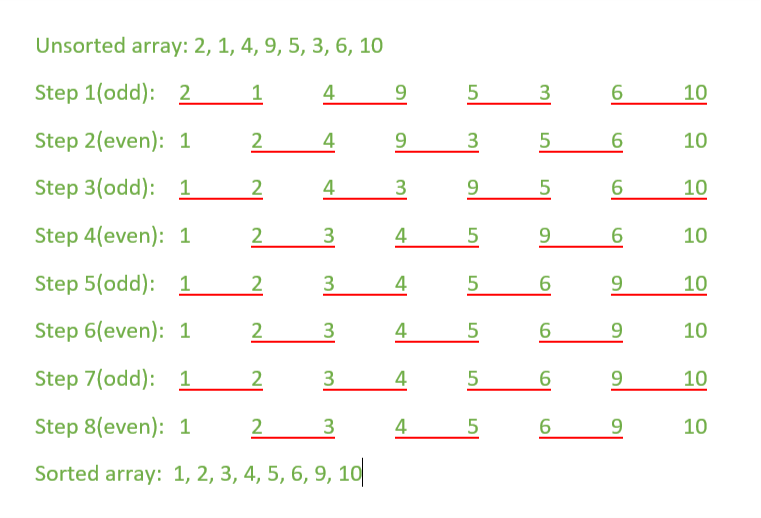# Odd Even Transposition Sort / Brick Sort using pthreads

Odd-Even Transposition Sort is a parallel sorting algorithm. It is based on the Bubble Sort technique, which compares every 2 consecutive numbers in the array and swap them if first is greater than the second to get an ascending order array. It consists of 2 phases – the odd phase and even phase:

• Odd phase: Every odd indexed element is compared with the next even indexed element(considering 1-based indexing).
• Even phase: Every even indexed element is compared with the next odd indexed element.

This article uses the concept of multi-threading, specifically pthread. In each iteration, every pair of 2 consecutive elements is compared using individual threads executing in parallel as illustrated below.Examples:

```Input: { 2, 1, 4, 9, 5, 3, 6, 10 }
Output: 1, 2, 3, 4, 5, 6, 9, 10

Input: { 11, 19, 4, 20, 1, 22, 25, 8}
Output: 1, 4, 8, 11, 19, 20, 22, 25
```

Note: Compile the program using following command on your Linux based system.

```g++ program_name.cpp -pthread
```

Below is the implementation of the above topic:

 `// CPP Program for Odd-Even Transpostion sort ` `// using pthreads ` ` `  `#include ` `#include ` ` `  `using` `namespace` `std; ` ` `  `// size of array ` `#define n 8 ` ` `  `// maximum number of threads ` `int` `max_threads = (n + 1) / 2; ` ` `  `int` `a[] = { 2, 1, 4, 9, 5, 3, 6, 10 }; ` `int` `tmp; ` ` `  `// Function to compare and exchange ` `// the consecutive elements of the array ` `void``* compare(``void``* arg) ` `{ ` ` `  `    ``// Each thread compares ` `    ``// two consecutive elements of the array ` `    ``int` `index = tmp; ` `    ``tmp = tmp + 2; ` ` `  `    ``if` `((a[index] > a[index + 1]) && (index + 1 < n)) { ` `        ``swap(a[index], a[index + 1]); ` `    ``} ` `} ` ` `  `void` `oddEven(pthread_t threads[]) ` `{ ` `    ``int` `i, j; ` ` `  `    ``for` `(i = 1; i <= n; i++) { ` `        ``// Odd step ` `        ``if` `(i % 2 == 1) { ` `            ``tmp = 0; ` ` `  `            ``// Creating threads ` `            ``for` `(j = 0; j < max_threads; j++) ` `                ``pthread_create(&threads[j], NULL, compare, NULL); ` ` `  `            ``// joining threads i.e. waiting ` `            ``// for all the threads to complete ` `            ``for` `(j = 0; j < max_threads; j++) ` `                ``pthread_join(threads[j], NULL); ` `        ``} ` ` `  `        ``// Even step ` `        ``else` `{ ` `            ``tmp = 1; ` ` `  `            ``// Creating threads ` `            ``for` `(j = 0; j < max_threads - 1; j++) ` `                ``pthread_create(&threads[j], NULL, compare, NULL); ` ` `  `            ``// joining threads i.e. waiting ` `            ``// for all the threads to complete ` `            ``for` `(j = 0; j < max_threads - 1; j++) ` `                ``pthread_join(threads[j], NULL); ` `        ``} ` `    ``} ` `} ` ` `  `// Function to print an array ` `void` `printArray() ` `{ ` `    ``int` `i; ` `    ``for` `(i = 0; i < n; i++) ` `        ``cout << a[i] << ``" "``; ` `    ``cout << endl; ` `} ` ` `  `// Driver Code ` `int` `main() ` `{ ` ` `  `    ``pthread_t threads[max_threads]; ` ` `  `    ``cout << ``"Given array is: "``; ` `    ``printArray(); ` ` `  `    ``oddEven(threads); ` ` `  `    ``cout << ``"\nSorted array is: "``; ` `    ``printArray(); ` ` `  `    ``return` `0; ` `} `

Output:

```Given array is:  2 1 4 9 5 3 6 10
Sorted array is: 1 2 3 4 5 6 9 10
```

Time complexity: The time complexity is reduced to O(N) due to parallel computation using threads.
Work complexity: The work complexity of this program is O(N) as N/2 number of threads(resources) are being used to sort the array. So, the work-time complexity of the program is O(N^2).

Attention reader! Don’t stop learning now. Get hold of all the important DSA concepts with the DSA Self Paced Course at a student-friendly price and become industry ready.

My Personal Notes arrow_drop_upCheck out this Author's contributed articles.

If you like GeeksforGeeks and would like to contribute, you can also write an article using contribute.geeksforgeeks.org or mail your article to contribute@geeksforgeeks.org. See your article appearing on the GeeksforGeeks main page and help other Geeks.

Please Improve this article if you find anything incorrect by clicking on the "Improve Article" button below.

Article Tags :
Practice Tags :

4

Please write to us at contribute@geeksforgeeks.org to report any issue with the above content.